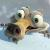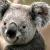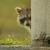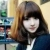## python 函数定义必须在调用前面吗

```def f1():
print('f1')
f1()```

```f1()

def f1():
print(f1)```

00python3里，方法都是先定义，后使用。

------------------------------------

# -*- encoding = utf-8 -*-

def foo1():
print("Hello foo1")

foo2()

def foo2():
print("Hello foo2")

if __name__ == '__main__':
foo1()

------------------------------------

Traceback (most recent call last):
File "py_func_def_926_ex.py", line 8, in <module>
foo2()
NameError: name 'foo2' is not defined

0Python只能先定义再调用。

``````def main(): #程序的主干
function1()
function2()

def function1():
....

def function2():
....

main()``````

``````try:
main()
except someError as e:
#异常处理代码
``````

0[python] view plain copy

1. Python 3.4.2 (v3.4.2:ab2c023a9432, Oct  6 2014, 22:16:31) [MSC v.1600 64 bit (AMD64)] on win32
2. Type "copyright", "credits" or "license()" for more information.
3. >>> def foo(p1, p2=6, p3):
4.     return 0
5. SyntaxError: non-default argument follows default argument
6. >>>

``````def 函数名(参数1，参数2，...,参数n):
函数体(语句块)``````

1.函数的命名规则要符合Python中命名要求，一般用小写字母和下划线、数字等组合
2.def是定义函数的关键词，这个简写来自英文单词define
3.函数后面是hi圆括号，可以有参数列表，也可以没有参数
4.括号后面的冒号不要忘记了
5.函数体（语句块），相对于def缩进四个空格

### 例子：

``````>>> def name():                 #定义一个无参数的函数，只是通过这个函数打印
...     print "python function" #函数体，缩进4个空格
...
>>> name()                      #调用函数，打印结果``````
``````>>> def add(x,y):       #定义一个加法函数
...     return x+y      #缩进4个空格
...
>>> add(3,4)            #通过函数计算3+4的和``````
``````>>> add("hello ","world")
'hello world'
>>>``````

``````>>> result = add(4,6)
>>> result
10``````

add(x,y)在运行之前，计算机内是不存在的，知道代码运行到这里的时候，在计算机中，就建立起来了一个对象，运行add(x,y)之后，也就建立了一个add(x,y)对象，这个对象与变量result可以建立引用关系，并且add(x,y)将计算结果返回。于是通过result可以查看运算结果。

### 参数的默认值

``````>>> def add_2(x,y=2):
...     return x+y
...
>>> add_2(2)
4
>>> add_2(4,4)
8``````
``````>>> def add_3(x=3,y):
...     return x+y
...
File "<stdin>", line 1
SyntaxError: non-default argument follows default argument``````
``````>>> def add_3(x=3,y=2):
...     return x+y
...
>>> add_3()                    #x = 3 , y = 2
5
>>>add_3(6,6)                  #x = 6 , y = 6
12``````
``````>>> def add(x,y=1,z):
...     return x+y+z
...
File "<stdin>", line 1
SyntaxError: non-default argument follows default argument``````
``````>>> def add(x,y=1,z=2):
...     return x+y+z
...
>>> add(2)        # x = 2 , y = 1 , z = 2
5``````

``def func(a,b = 1):  #有效``
``def func(a = 1,b):  #无效``